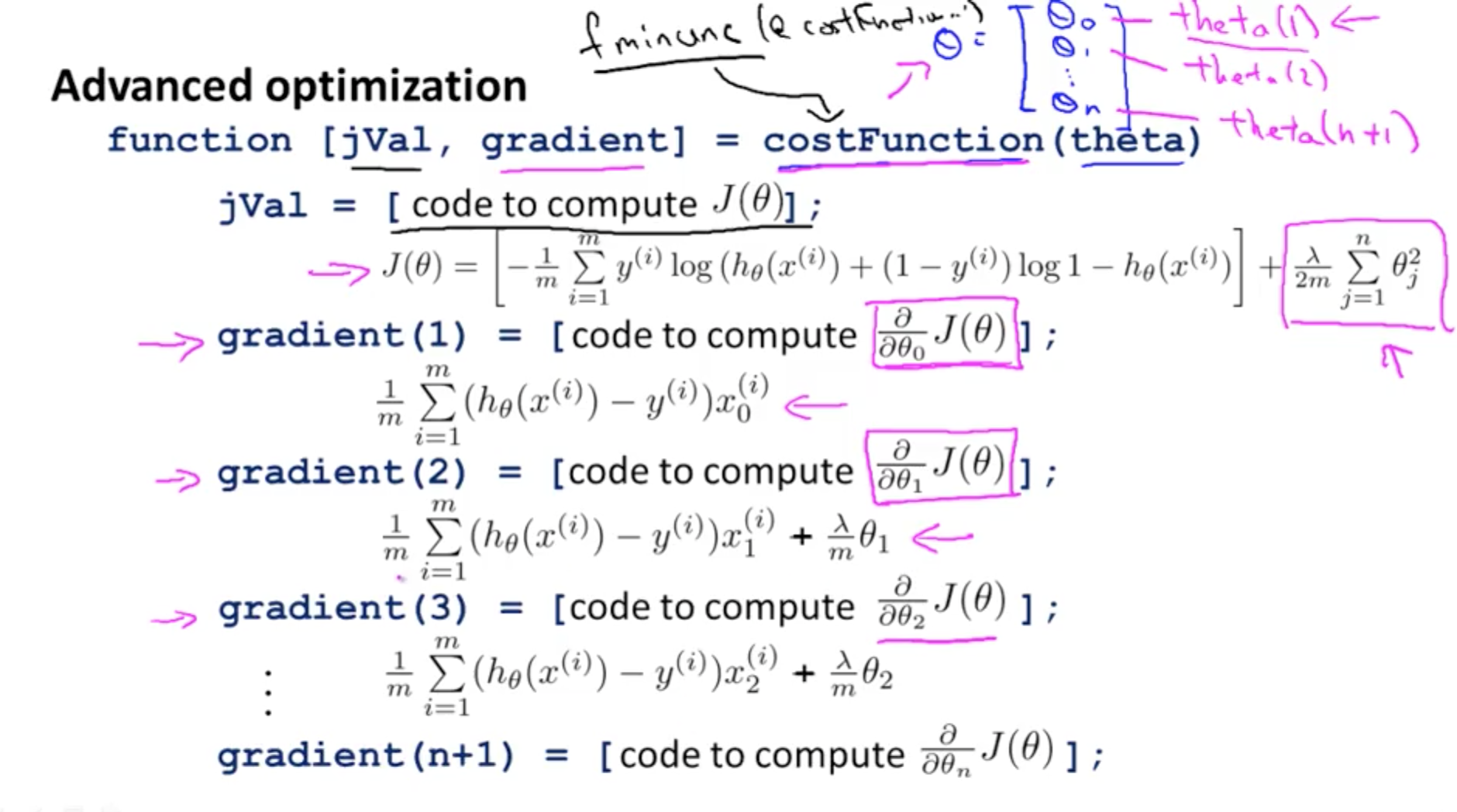Classification, logistic regression, advanced optimization, multi-class classification, overfitting, and regularization.

## 1. Classification and Representation

I would like to give full credits to the respective authors as these are my personal python notebooks taken from deep learning courses from Andrew Ng, Data School and Udemy :) This is a simple python notebook hosted generously through Github Pages that is on my main personal notes repository on https://github.com/ritchieng/ritchieng.github.io. They are meant for my personal review but I have open-source my repository of personal notes as a lot of people found it useful.

### 1a. Classification

• y variable (binary classification)
• 0: negative class
• 1: positive class
• Examples
• Email: spam / not spam
• Online transactions: fraudulent / not fraudulent
• Tumor: malignant / not malignant
• Issue 1 of Linear Regression
• As you can see on the graph, your prediction would leave out malignant tumors as the gradient becomes less steep with an additional data point on the extreme right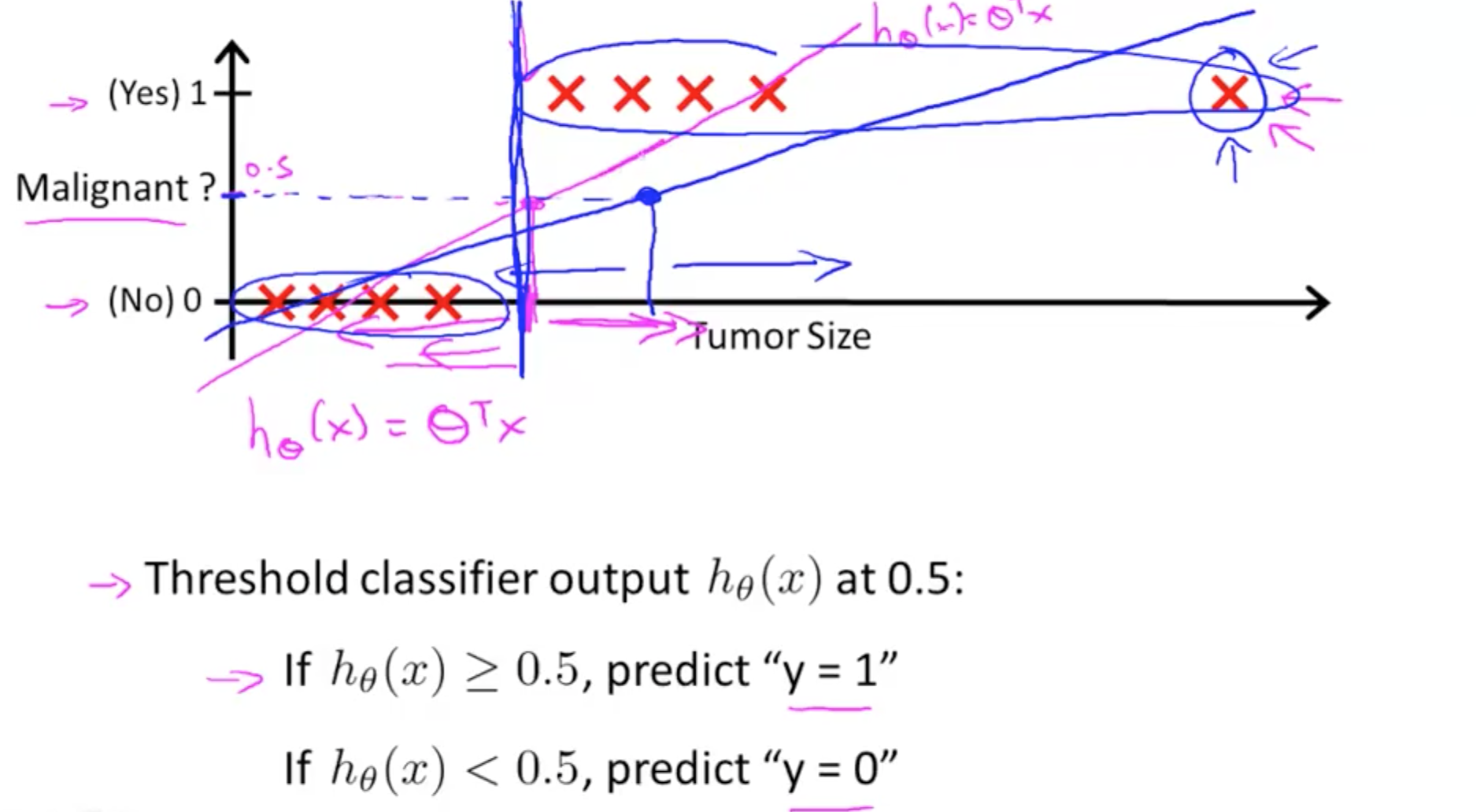• Issue 2 of Linear Regression
• Hypothesis can be larger than 1 or smaller than zero
• Hence, we have to use logistic regression

## 1b. Logistic Regression Hypothesis

• Logistic Regression Model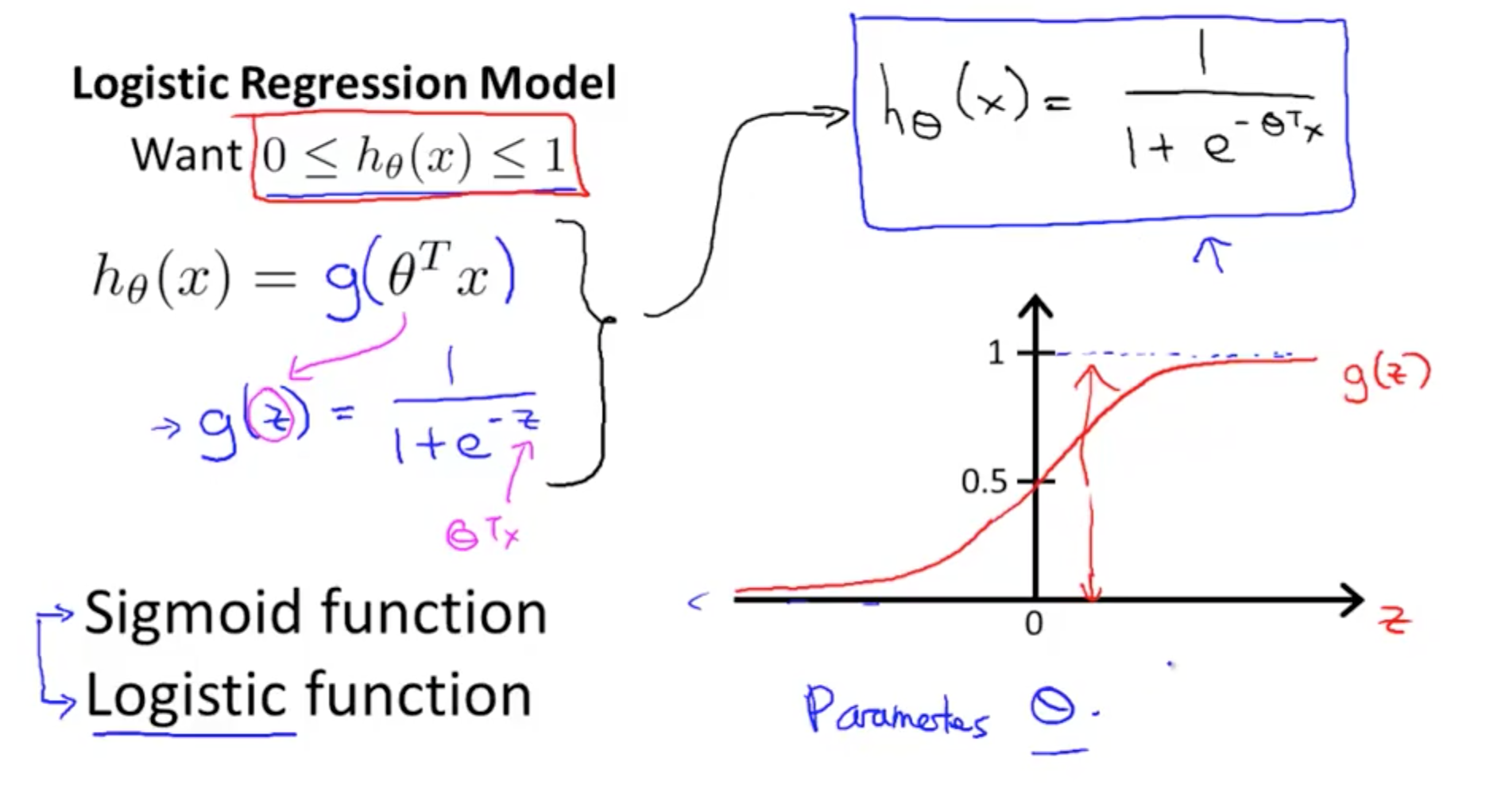• Interpretation of Hypothesis Output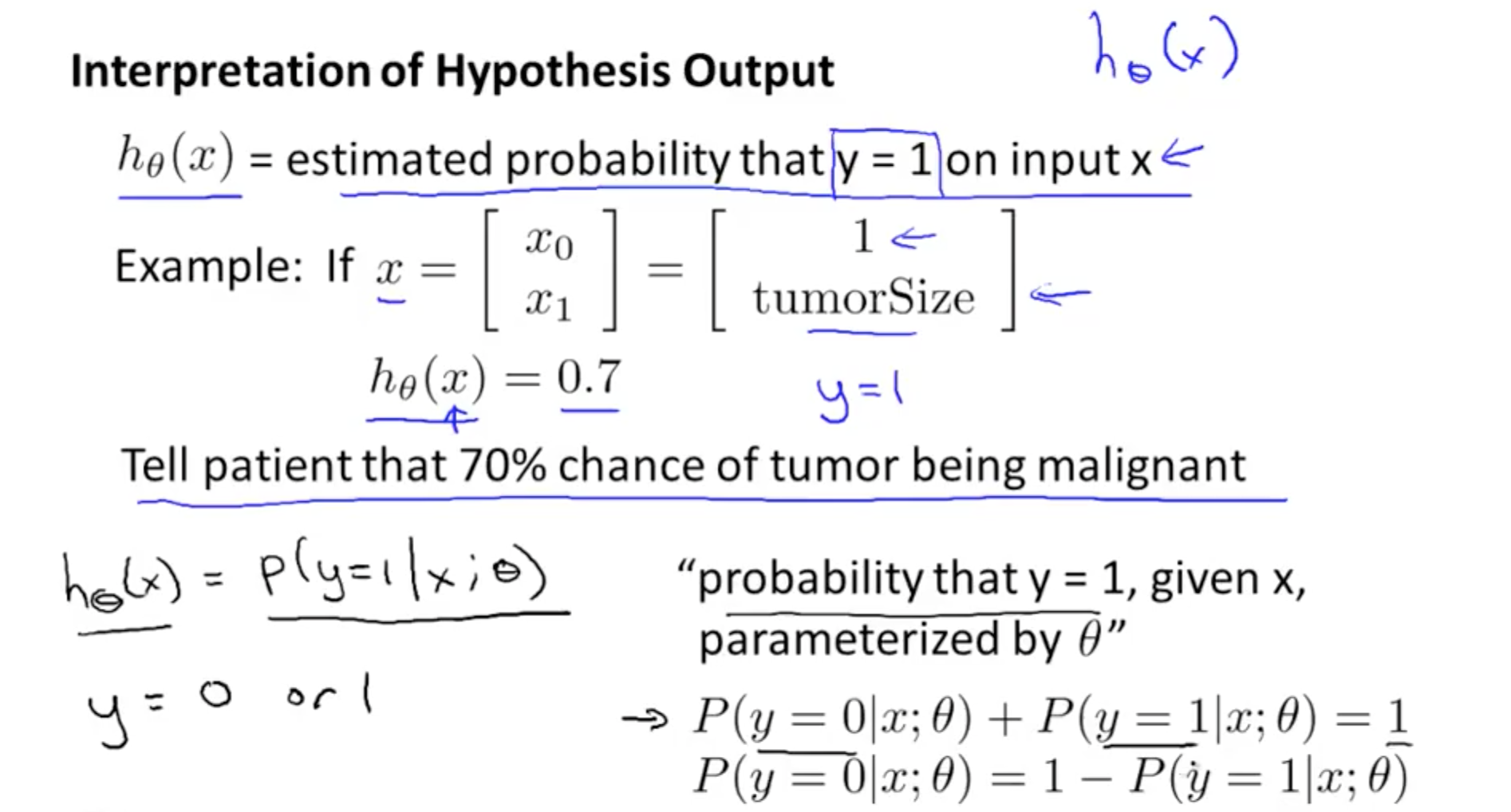## 1c. Decision Boundary

• Boundaries
• Max 1
• Min 0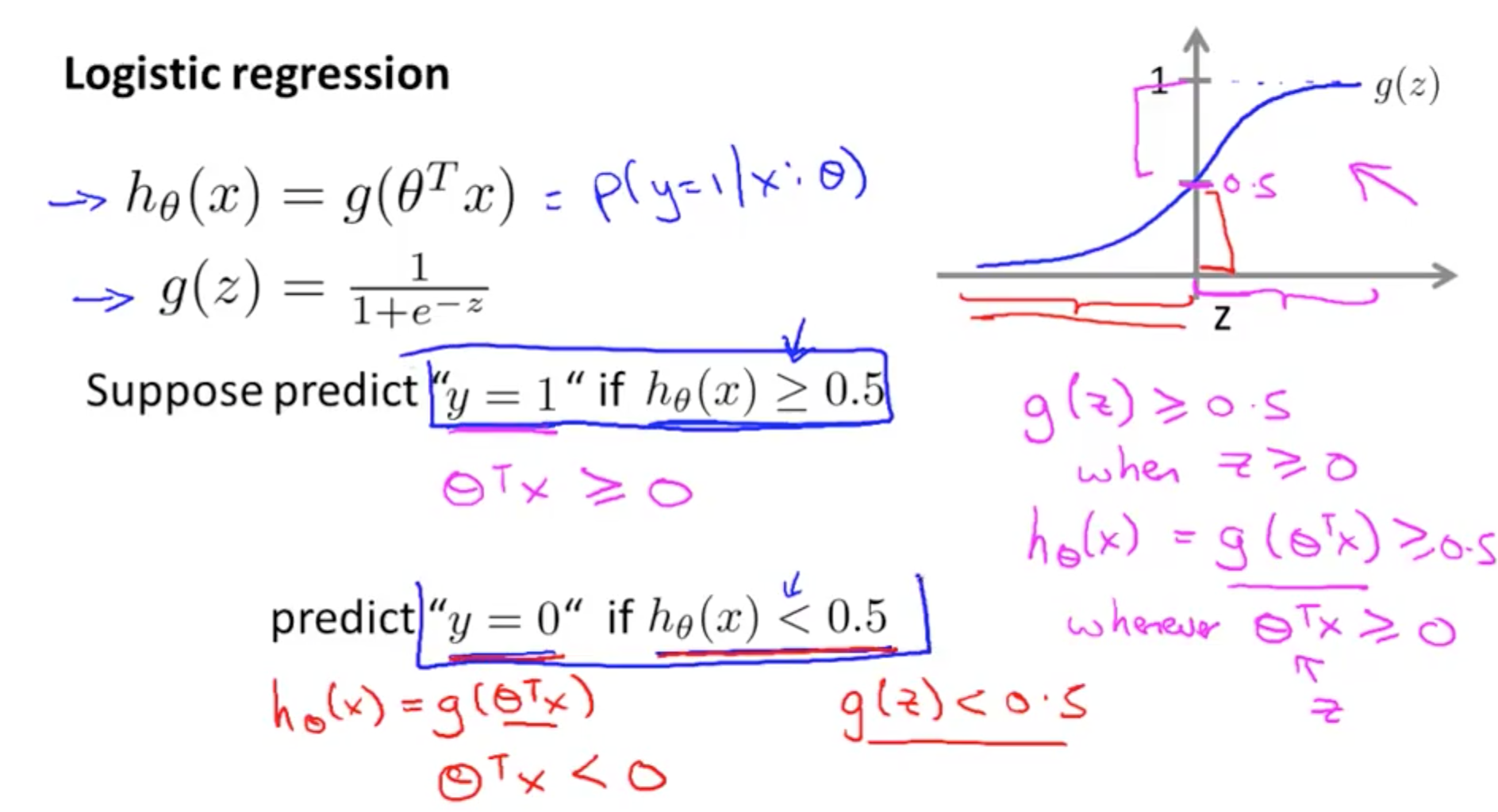• Boundaries are properties of the hypothesis not the data set
• You do not need to plot the data set to get the boundaries
• This will be discussed subsequently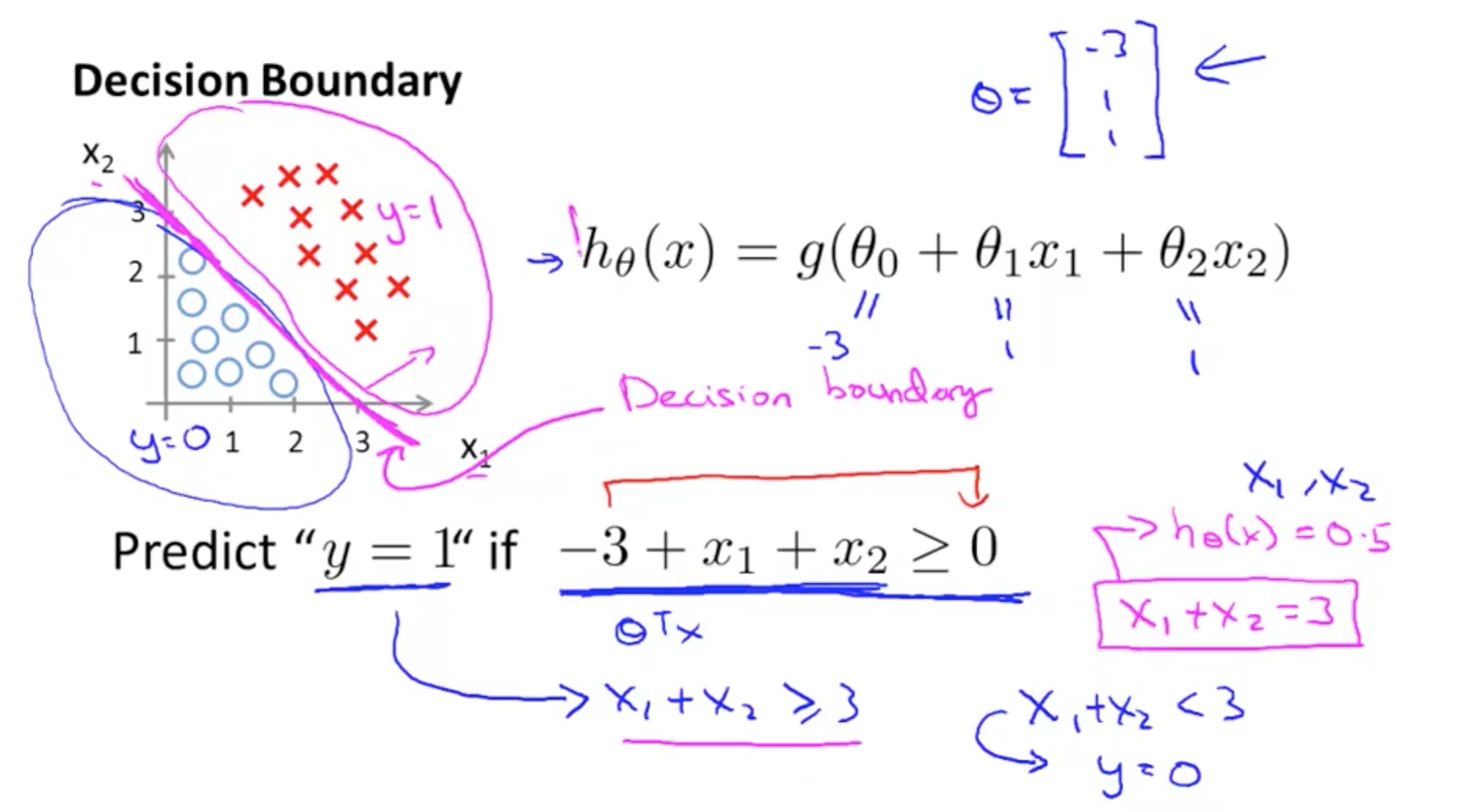• Non-linear decision boundaries
• Add higher order polynomial terms as features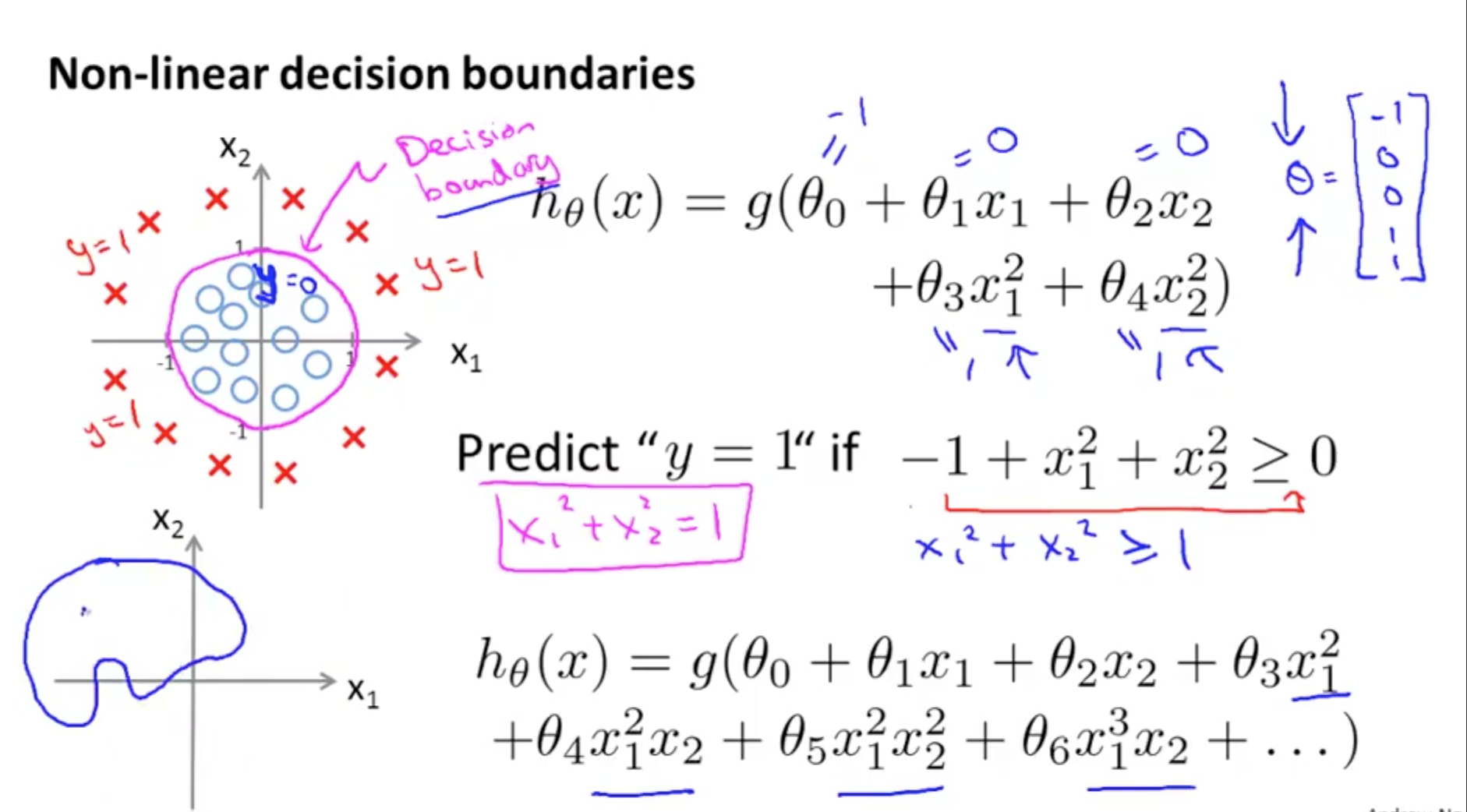## 2. Logistic Regression Model

### 2a. Cost Function

• How do we choose parameters?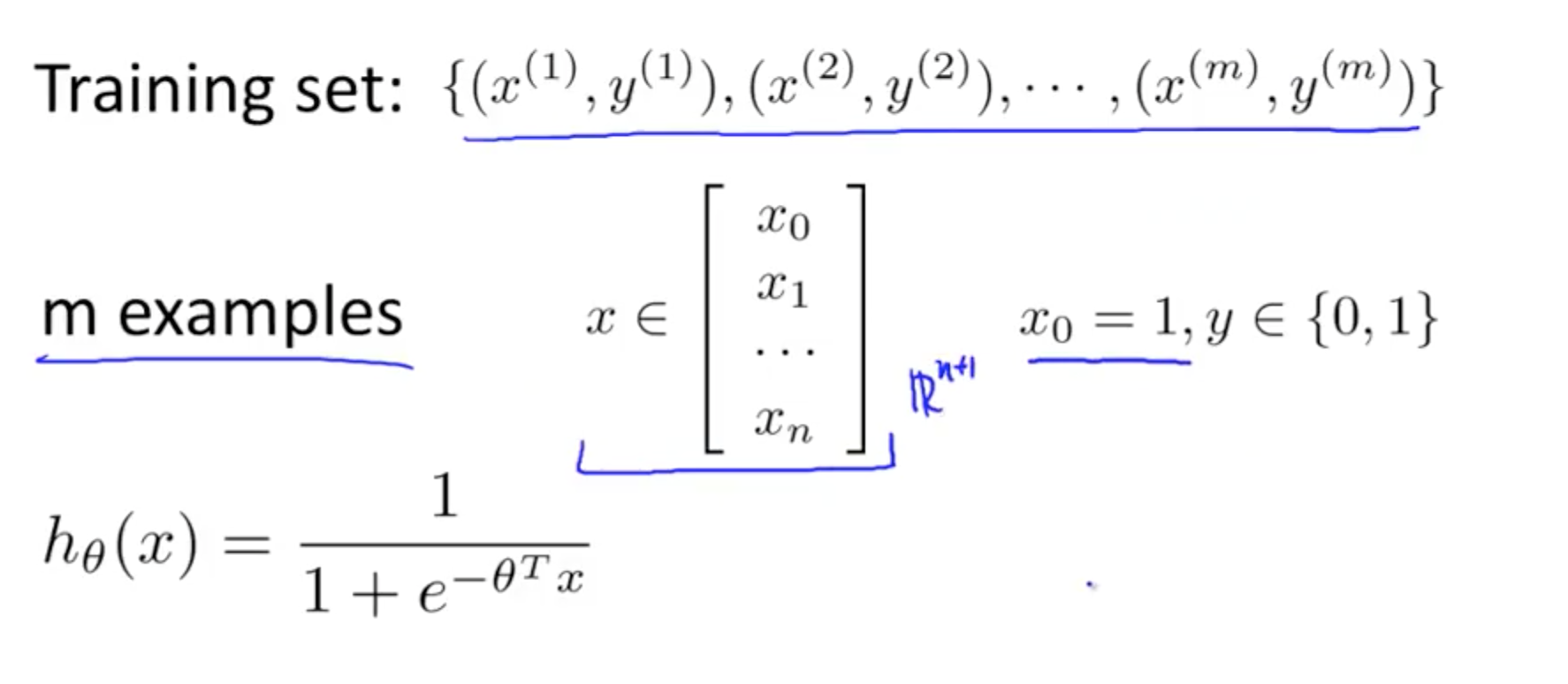• If y = 1
• If h(x) = 0 & y = 1, costs infinite
• If h(x) = 1 & y = 1 , costs = 0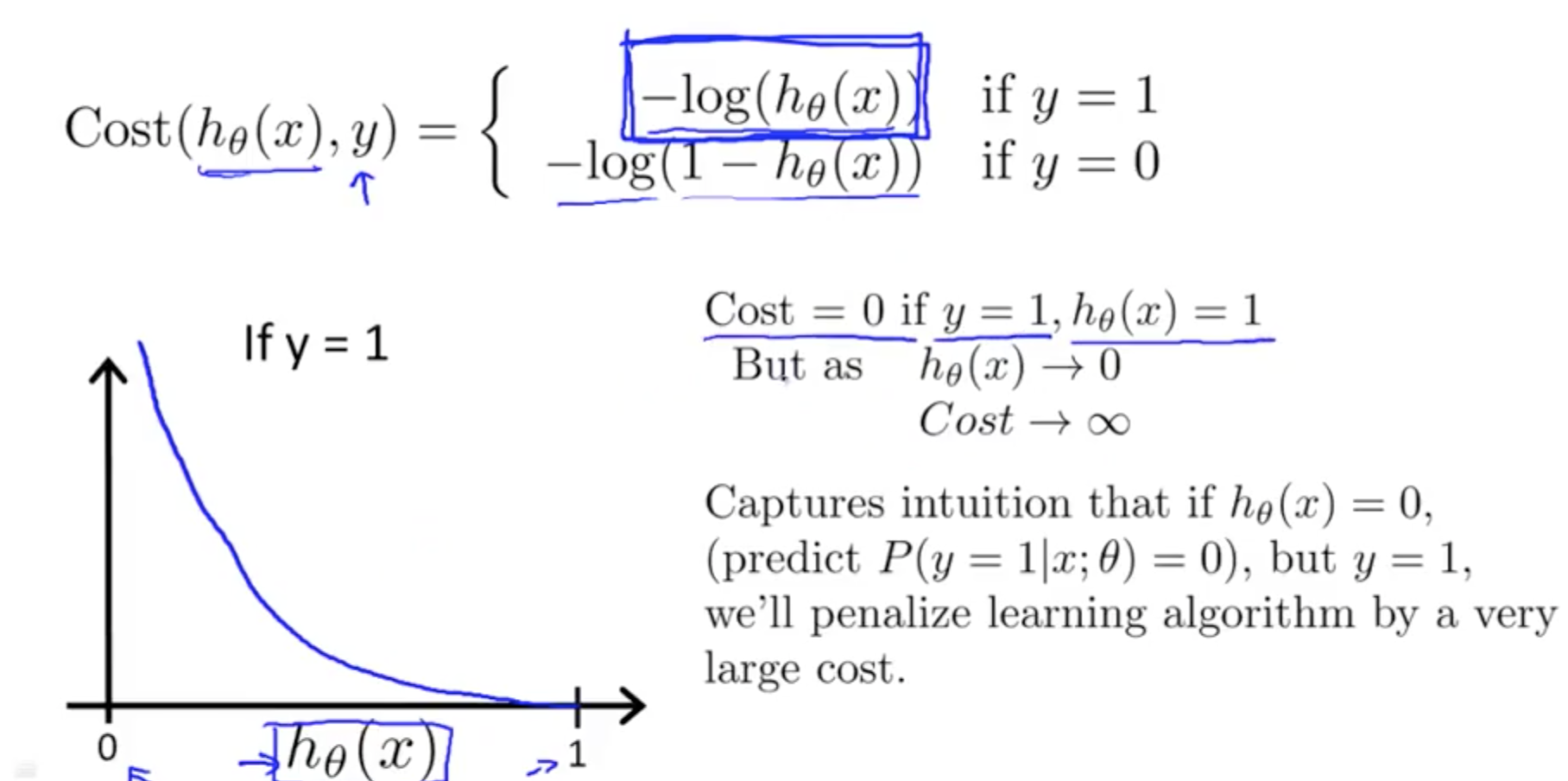• If y = 0
• If h(x) = 0 & y = 0, costs = 0
• If h(x) = 1 & y = 0, costs infinite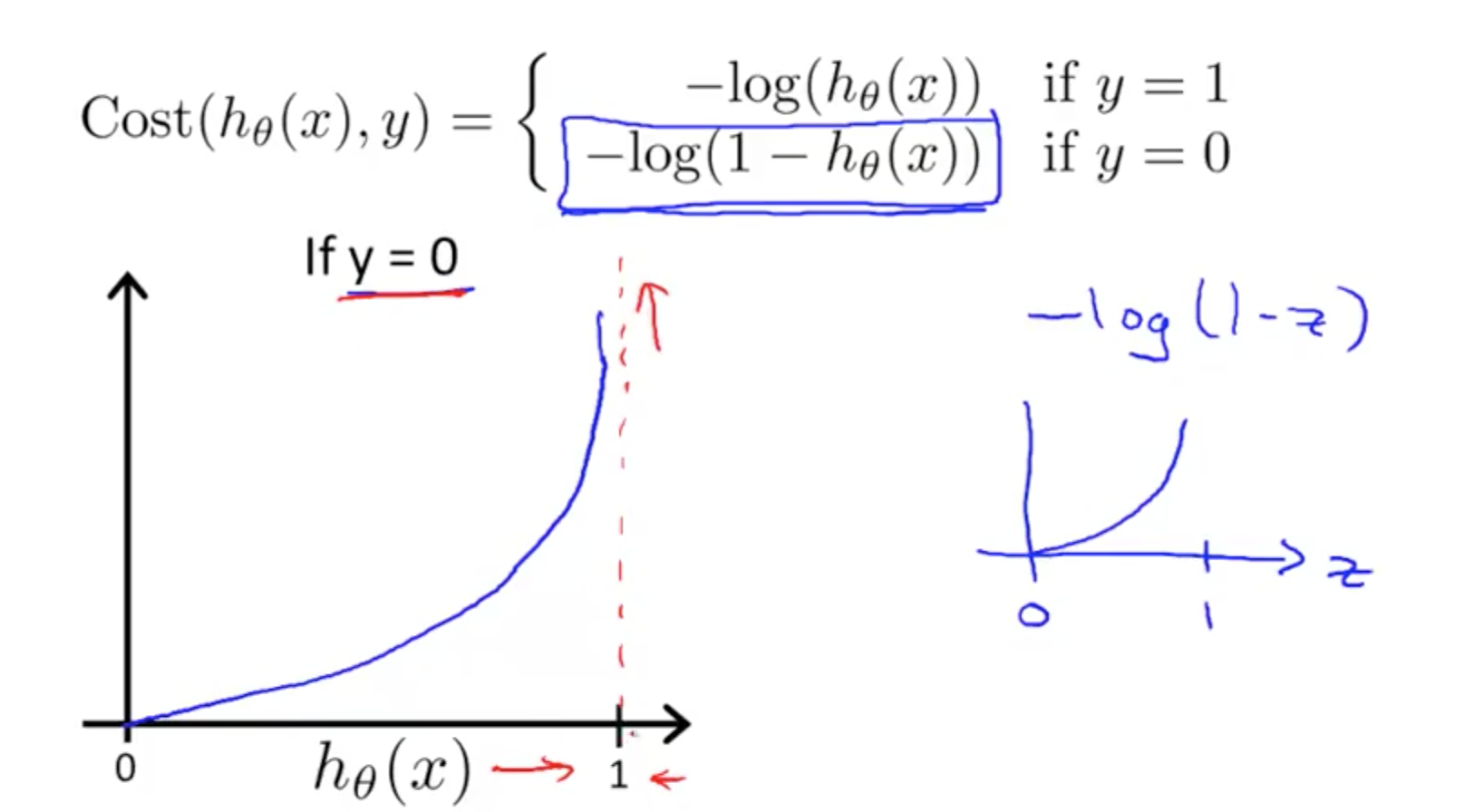### 2b. Simplified Cost Function & Gradient Descent

• Simplified Cost Function Derivatation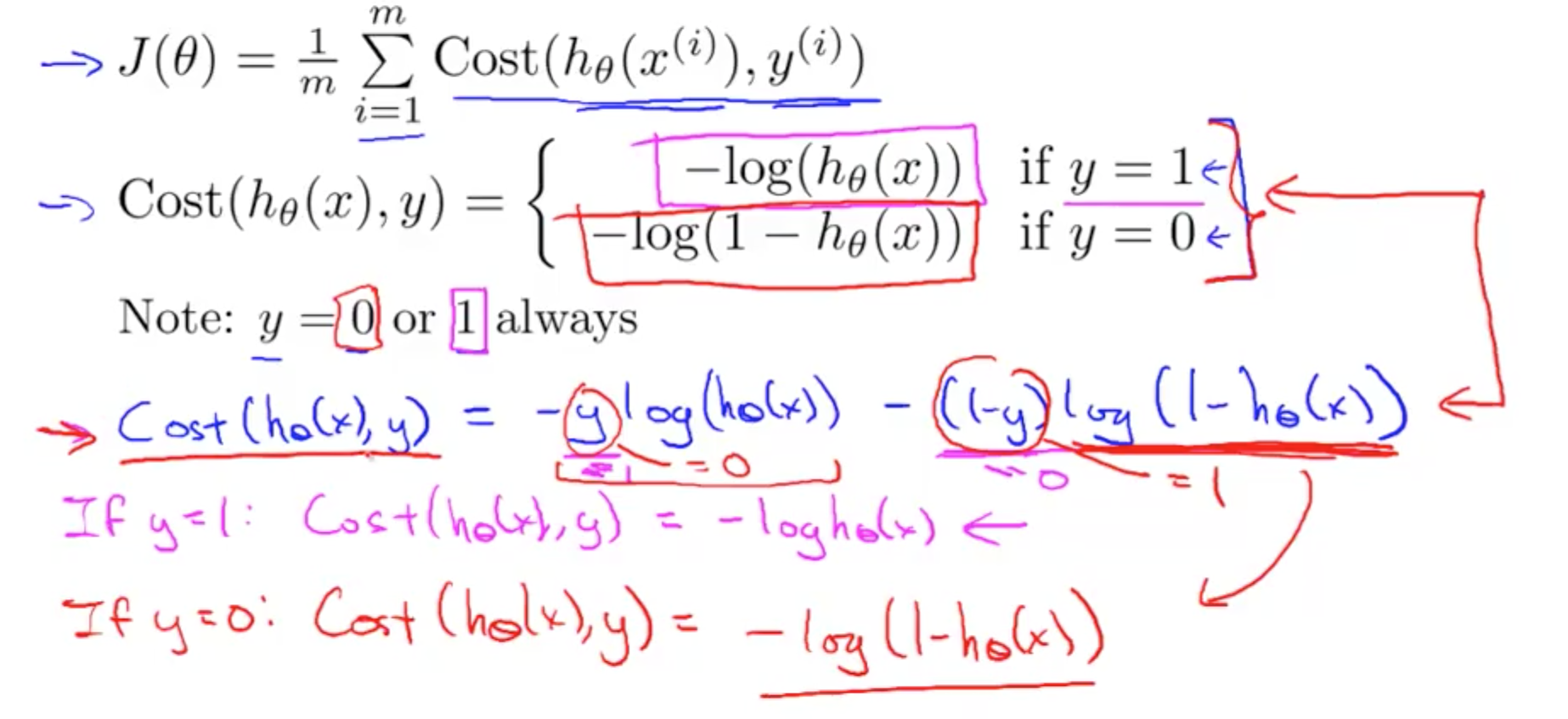• Simplified Cost Function
• Always convex so we will reach global minimum all the time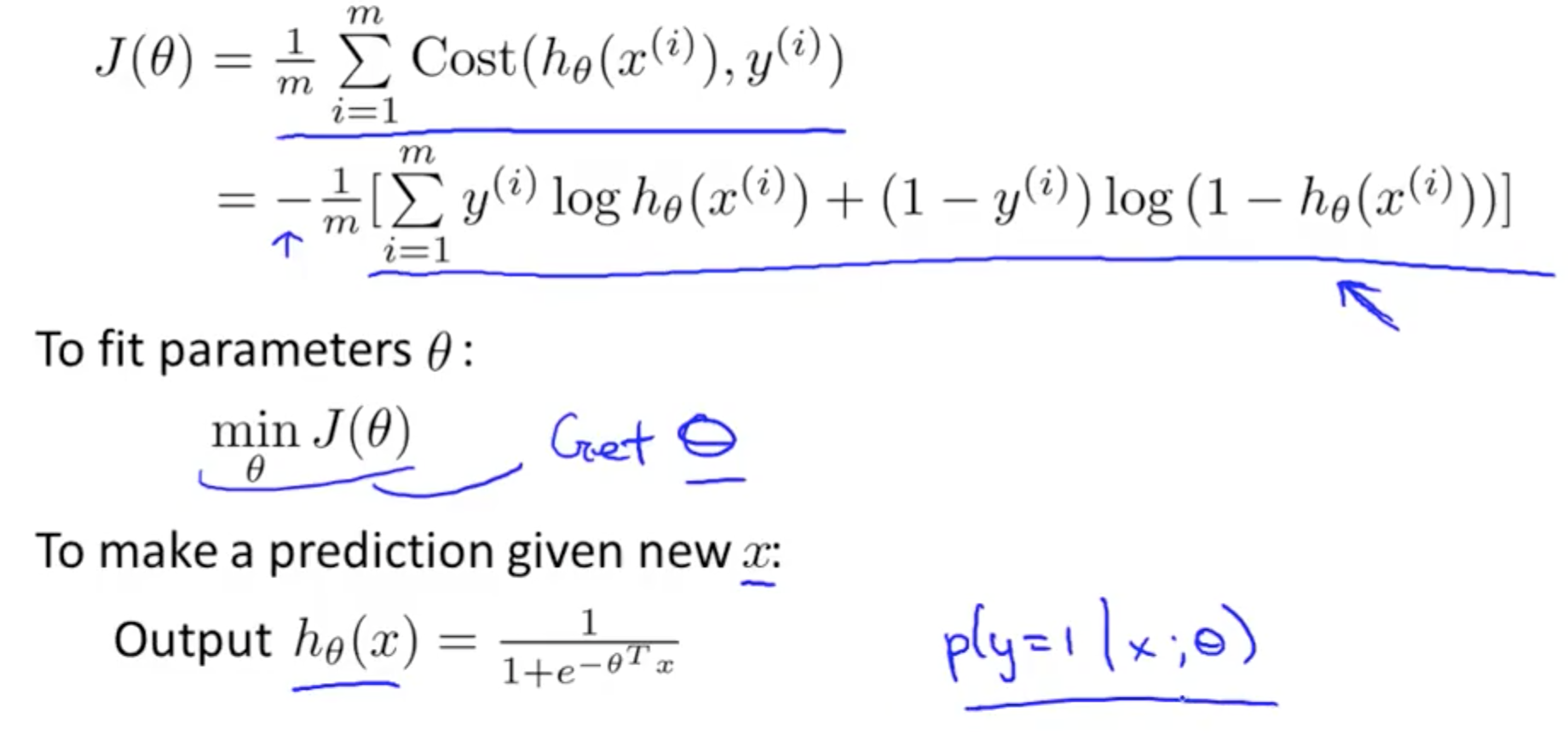• It looks identical, but the hypothesis for Logistic Regression is different from Linear Regression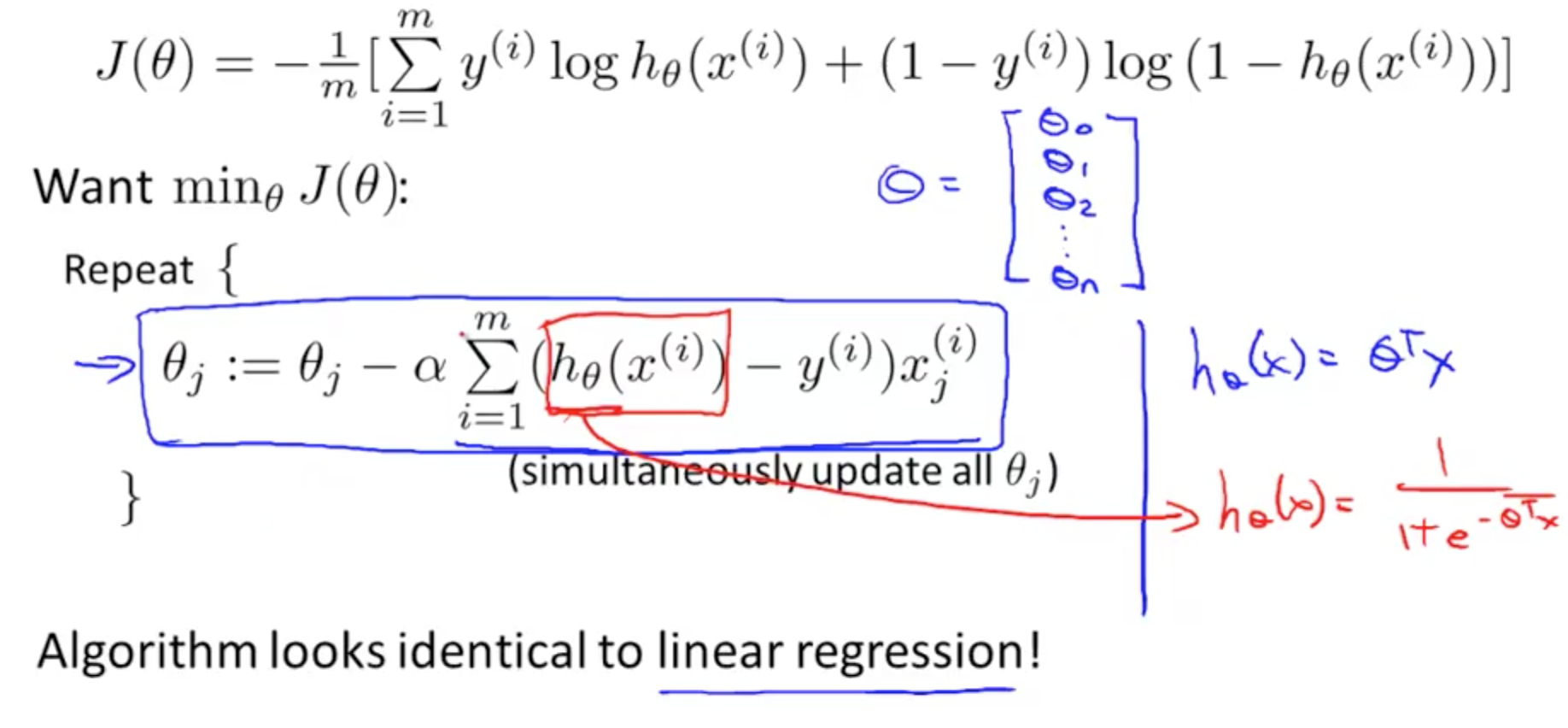• Ensuring Gradient Descent is Running Correctly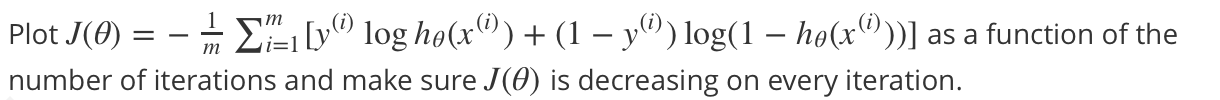• Background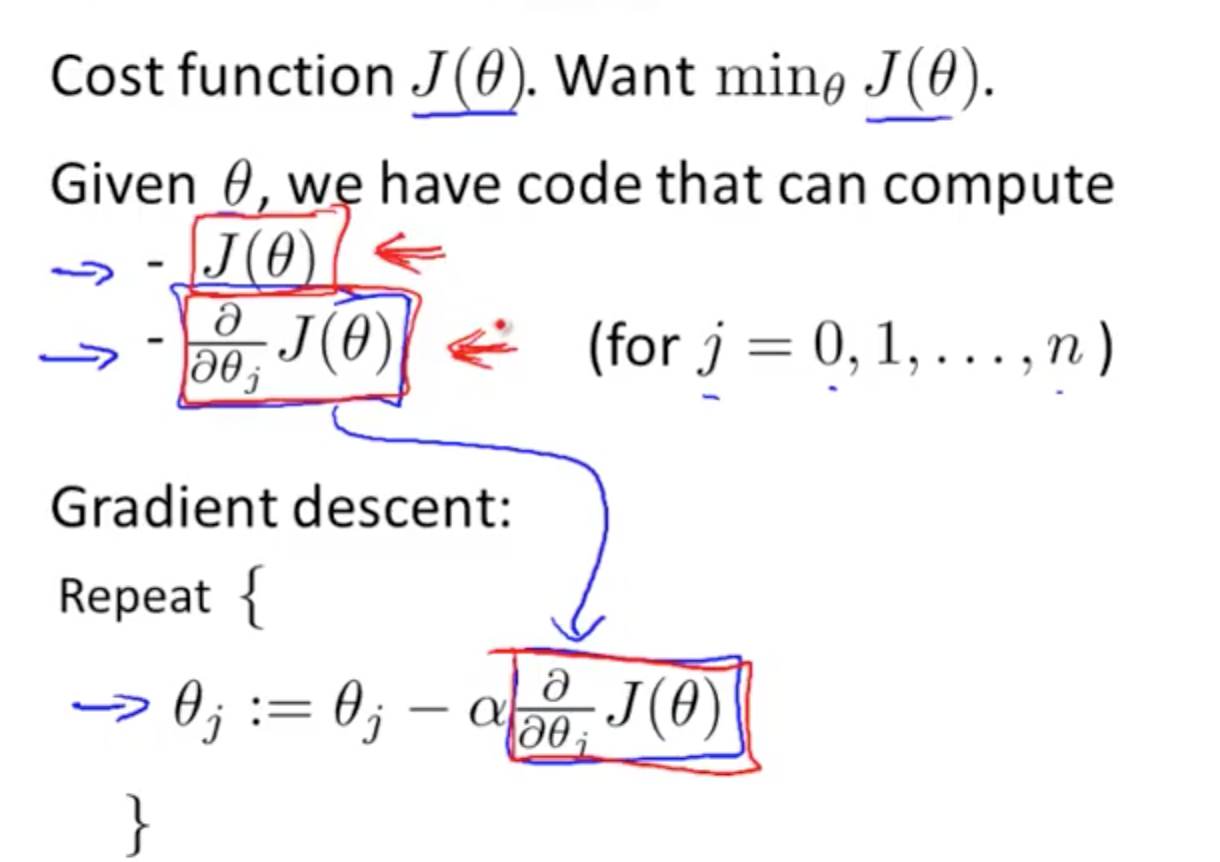• Optimization algorithm
• Others
• BFGS
• L-BFGS
• No need to manually pick alpha
• Often faster than gradient descent
• More complex
• Should not implement these yourself unless you’re an expert in numerical computing
• Use a software library to do them
• There are good and bad implementations, choose wisely
• Components of code explanation
• Code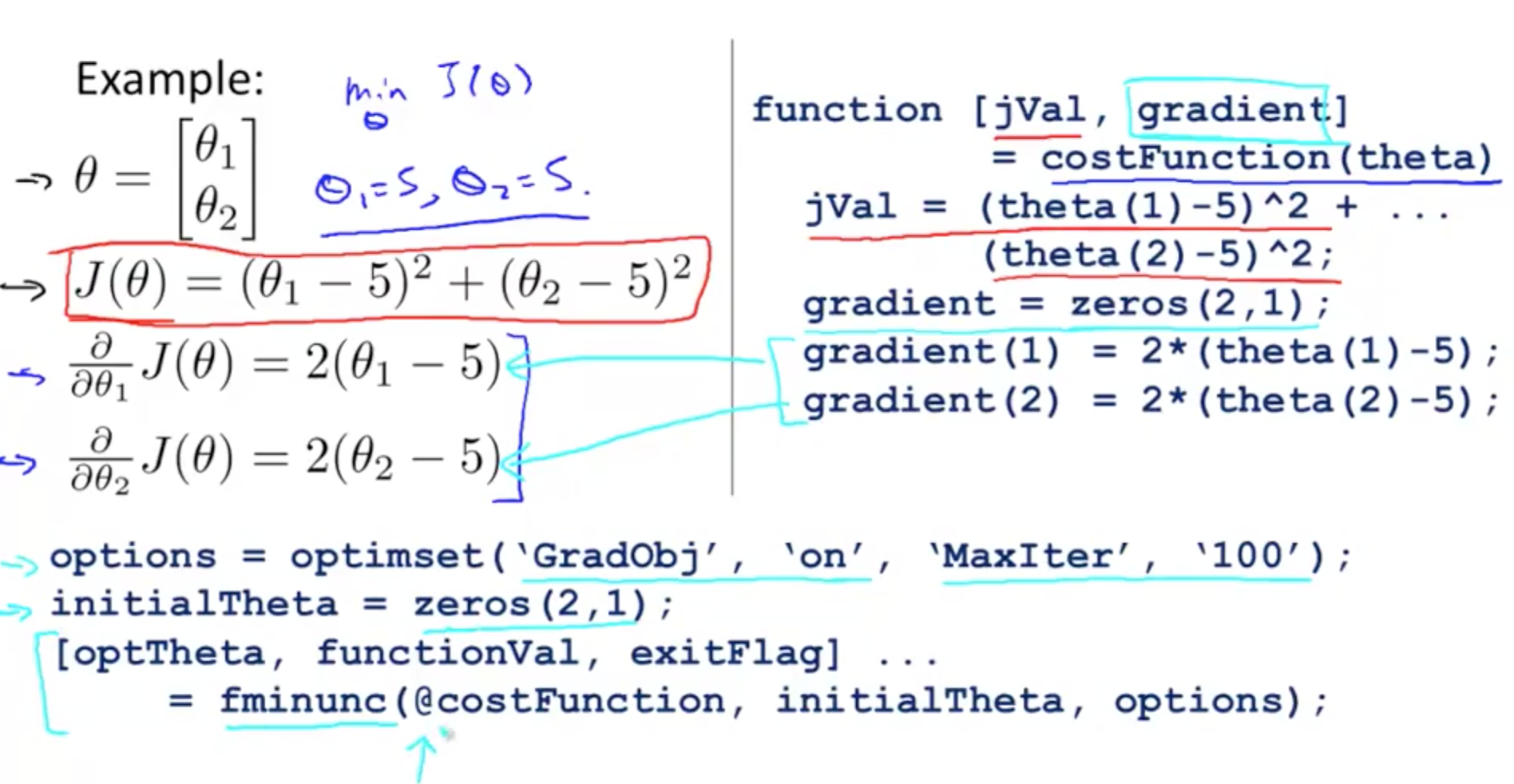• We will be providing gradient to this algorithm
• ‘MaxIter’, ‘100’
• Max iterations to 100
• fminunc
• Function minimisation unconstrained
• Cost minimisation function in octave
• @costFunction
• Points to our defined function
• optTheta
• Automatically choose learning rate
• Results
• Theta0 = 5
• Theta1 = 5
• functionVal = 1.5777e-030
• Essentially 0 for J(theta), what we are hoping for
• exitFlag = 1
• Verify if it has converged, 1 = converged
• Theta must be more than 2 dimensions
• Main point is to write a function that returns J(theta) and gradient to apply to logistic or linear regression
•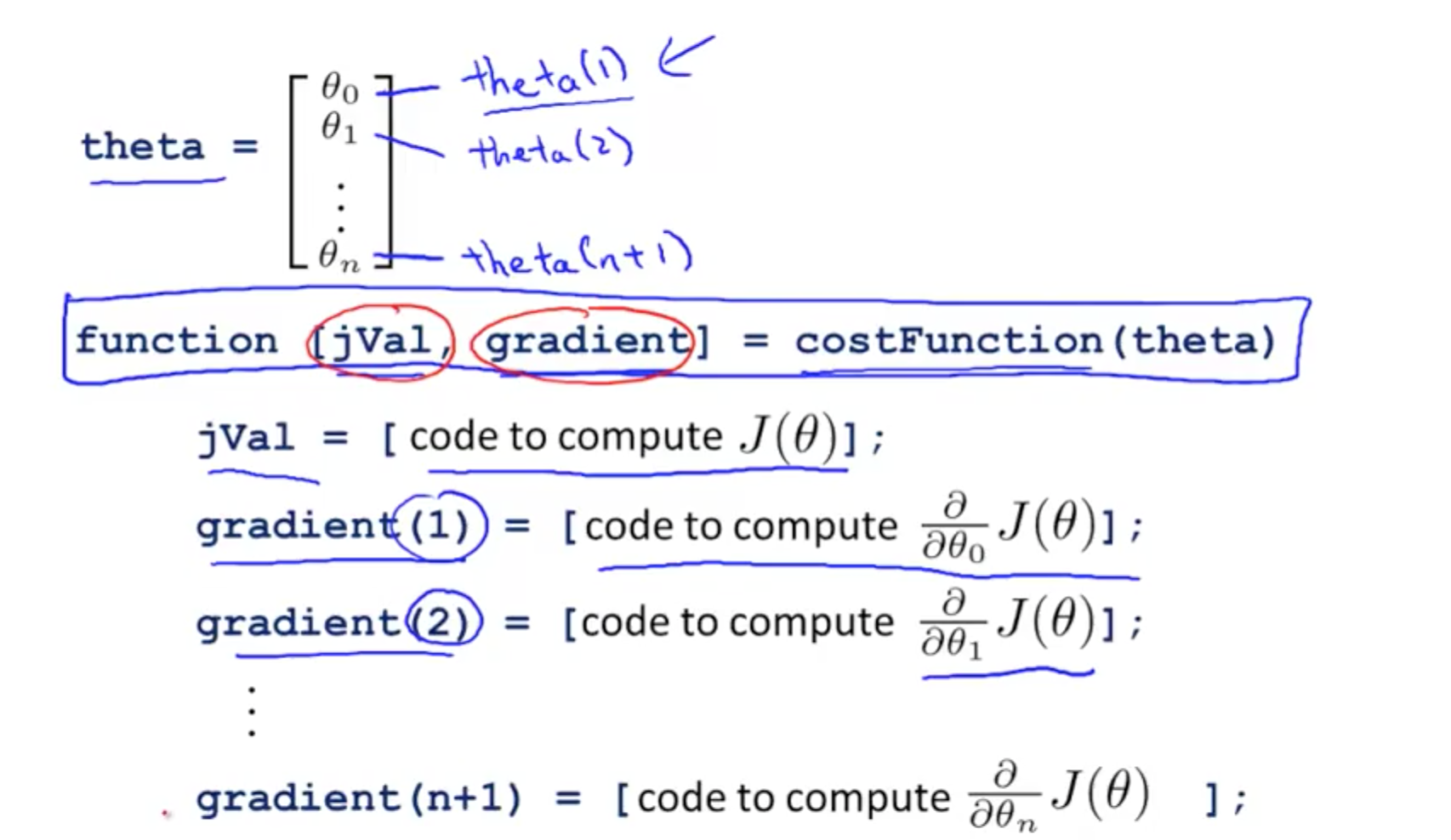## 3. Multi-class Classification

• Similar terms
• One-vs-all
• One-vs-rest
• Examples
• Email folders or tags (4 classes)
• Work
• Friends
• Family
• Hobby
• Medical Diagnosis (3 classes)
• Not ill
• Cold
• Flu
• Weather (4 classes)
• Sunny
• Cloudy
• Rainy
• Snow
• Binary vs Multi-class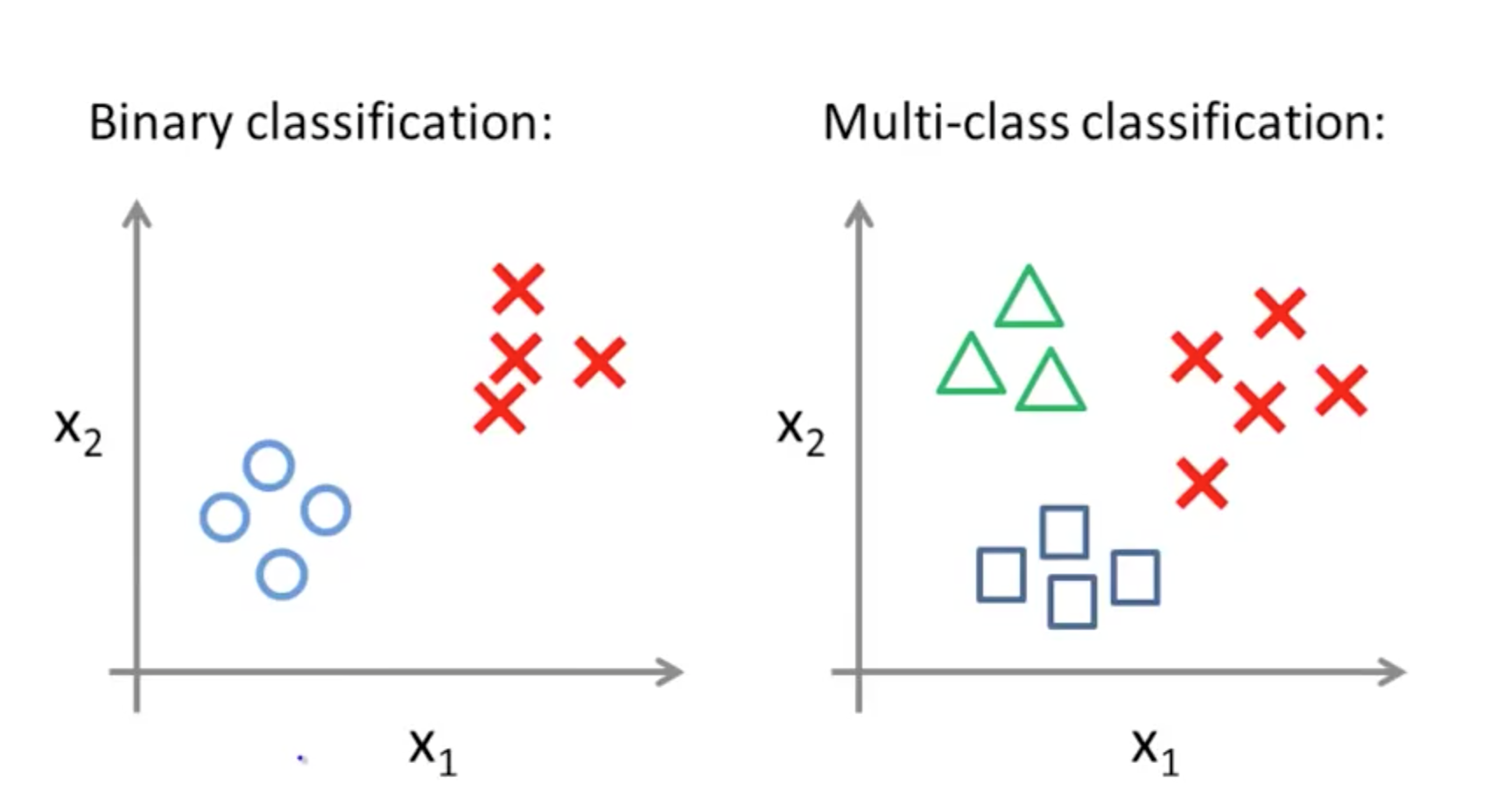• One-vs-all (One-vs-rest)
• Split them into 3 distinct groups and compare them to the rest
• If you have k classes, you need to train k logistic regression classifiers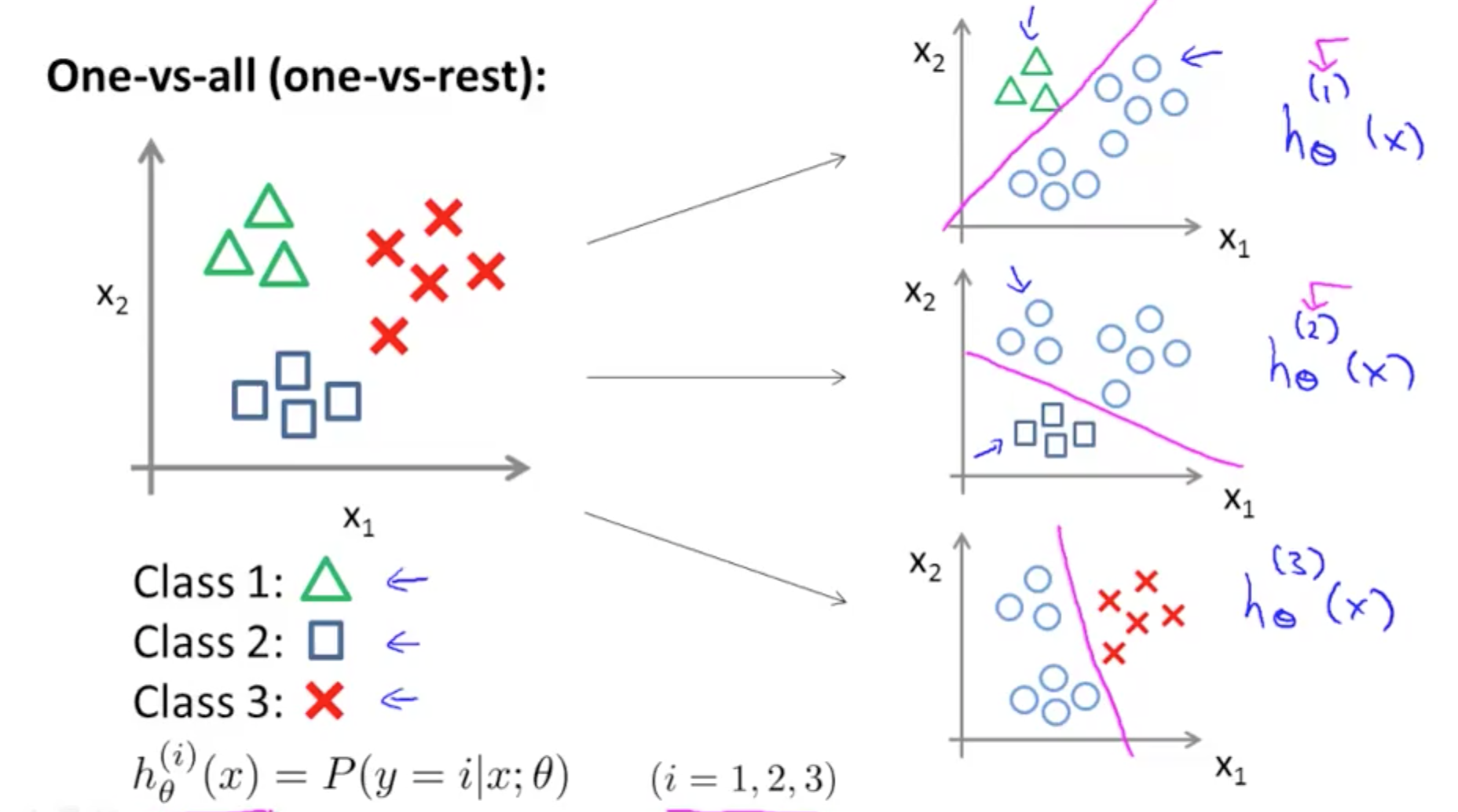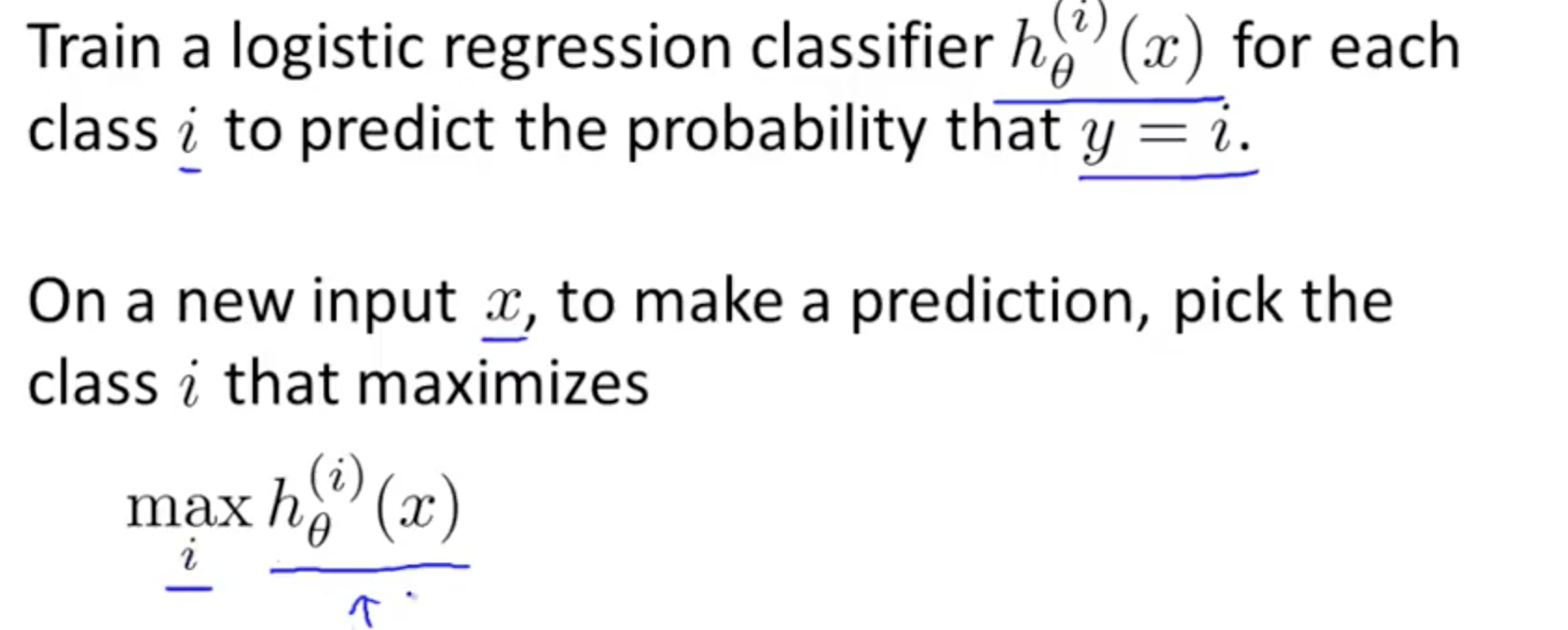## 4. Solving Problem of Overfitting

### 4a. Problem of Overfitting

• Linear Regression: Overfitting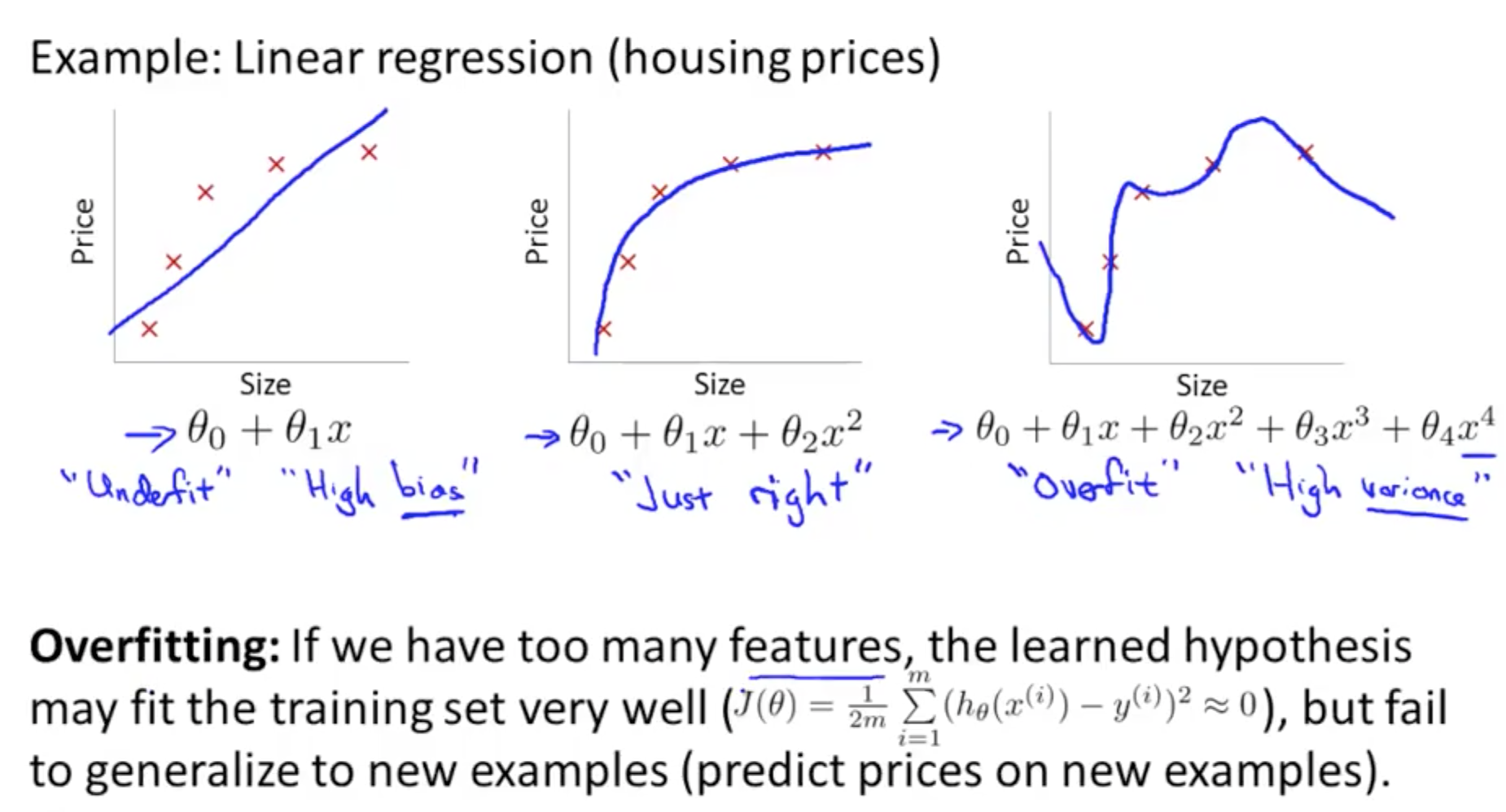• Overfit
• High Variance
• Too many features
• Fit well but fail to generalize new examples
• Underfit
• High Bias
• Logistic Regression: Overfitting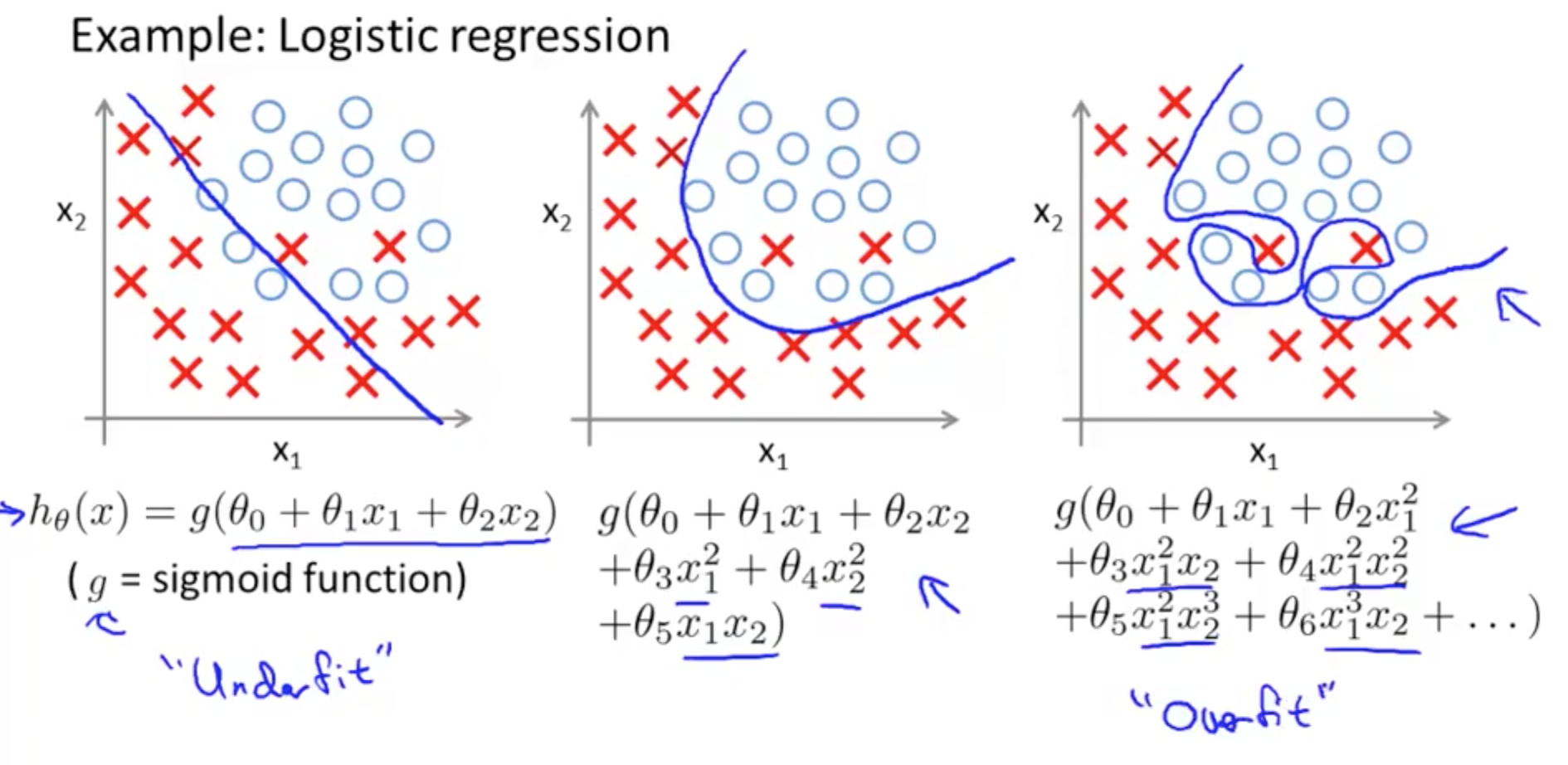• Solutions to Overfitting
• Reduce number of features
• Manually select features to keep
• Model selection algorithm
• Regularization
• Keep all features, but reduce magnitude or values of parameters theta_j
• Works well when we’ve a lot of features

### 4b. Cost Function

• Intuition
• Making theta so small that is almost equivalent to zero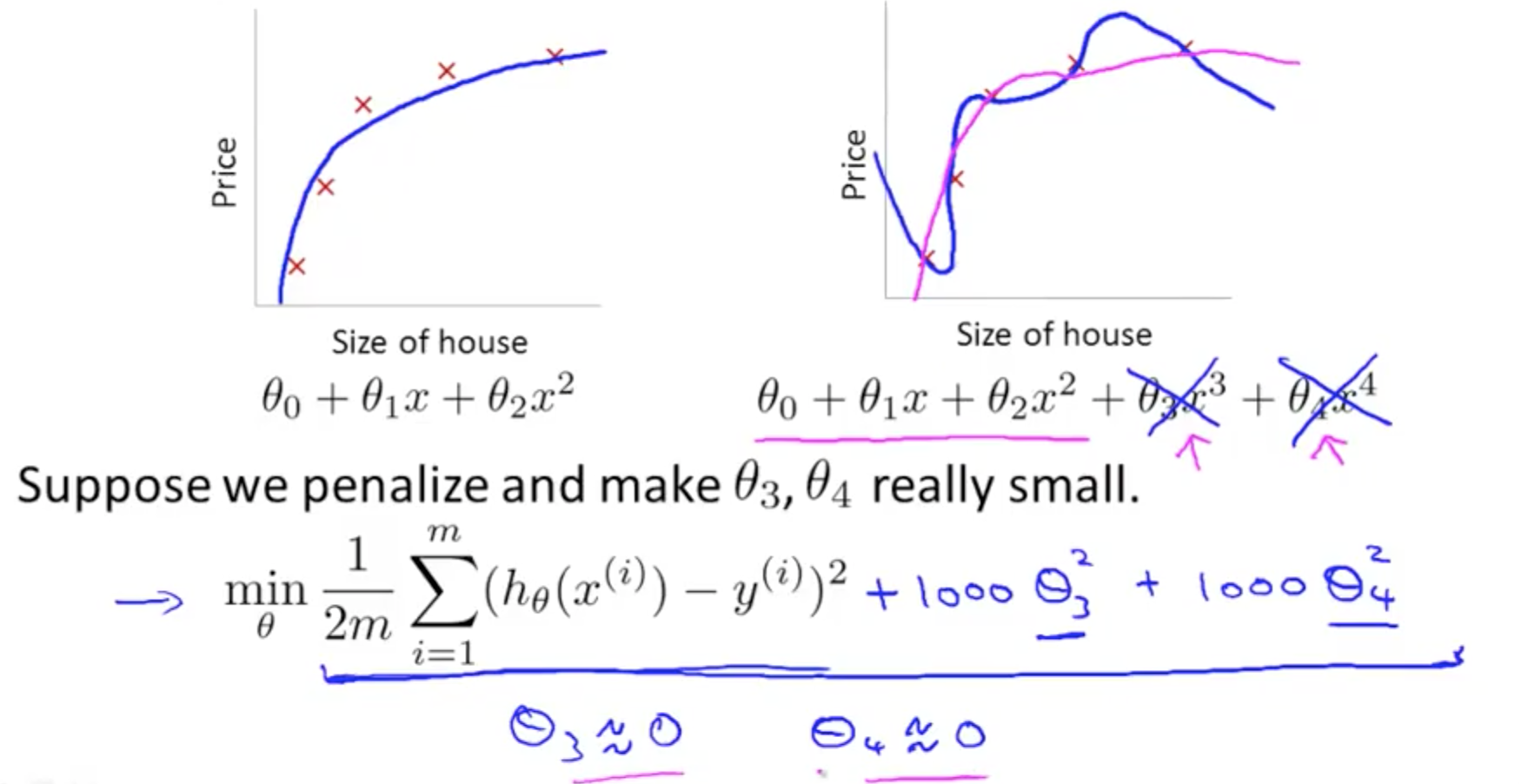• Regularization
• Small values for parameters (thetas)
• “Simpler” hypothesis
• Less prone to overfitting
• Add regularization parameter to J(theta) to shrink parameters
• First goal: fit training set well (first term)
• Second goal: keep parameter small (second, pink, term)
• If lamda is set to an extremely large value, this would result in underfitting
• High bias
• Only penalize thetas from 1, not from 0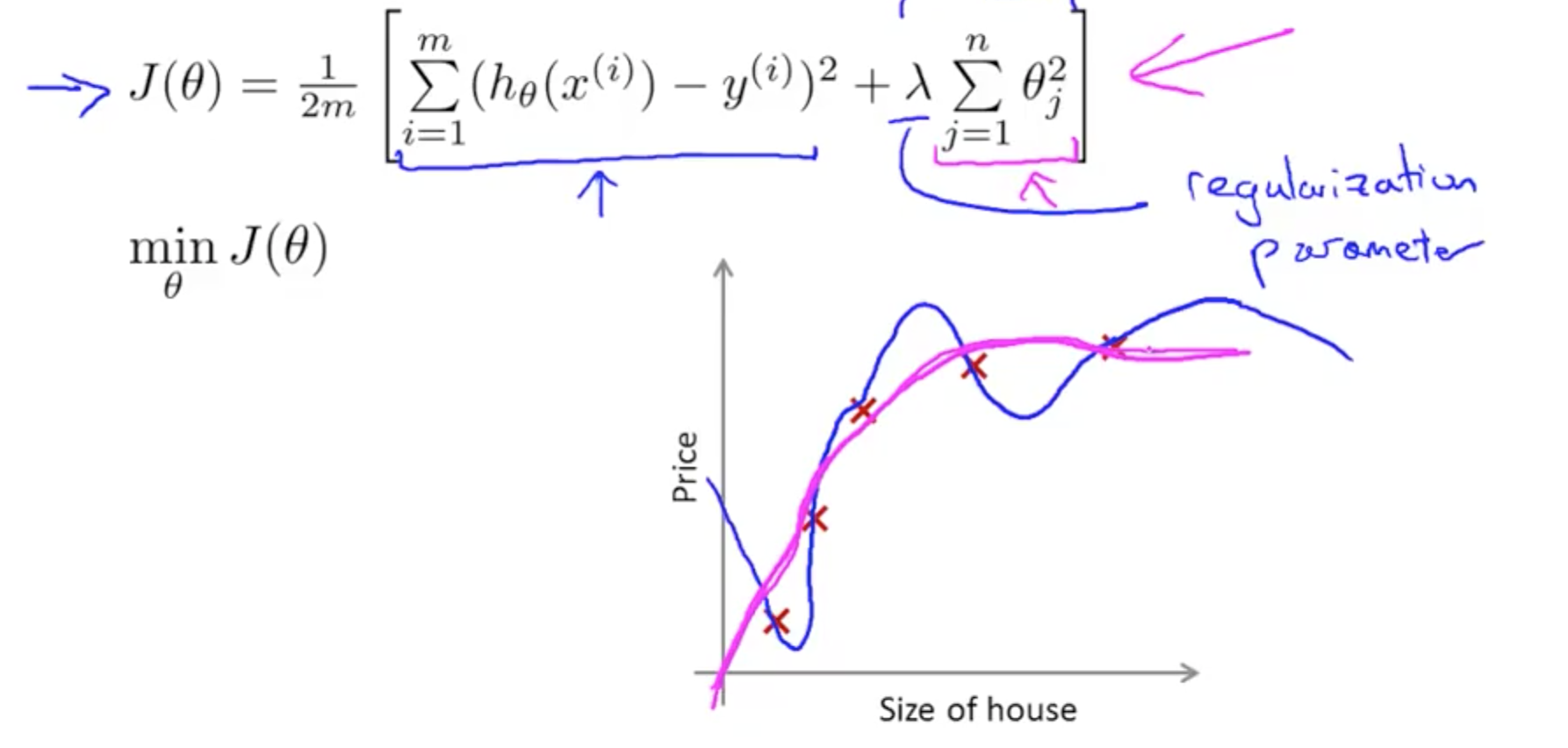### 4c. Regularized Linear Regression

• Usually, (1- alpha * lambda / m) is 0.99• Normal Equation
• Alternative to minimise J(theta) only for linear regression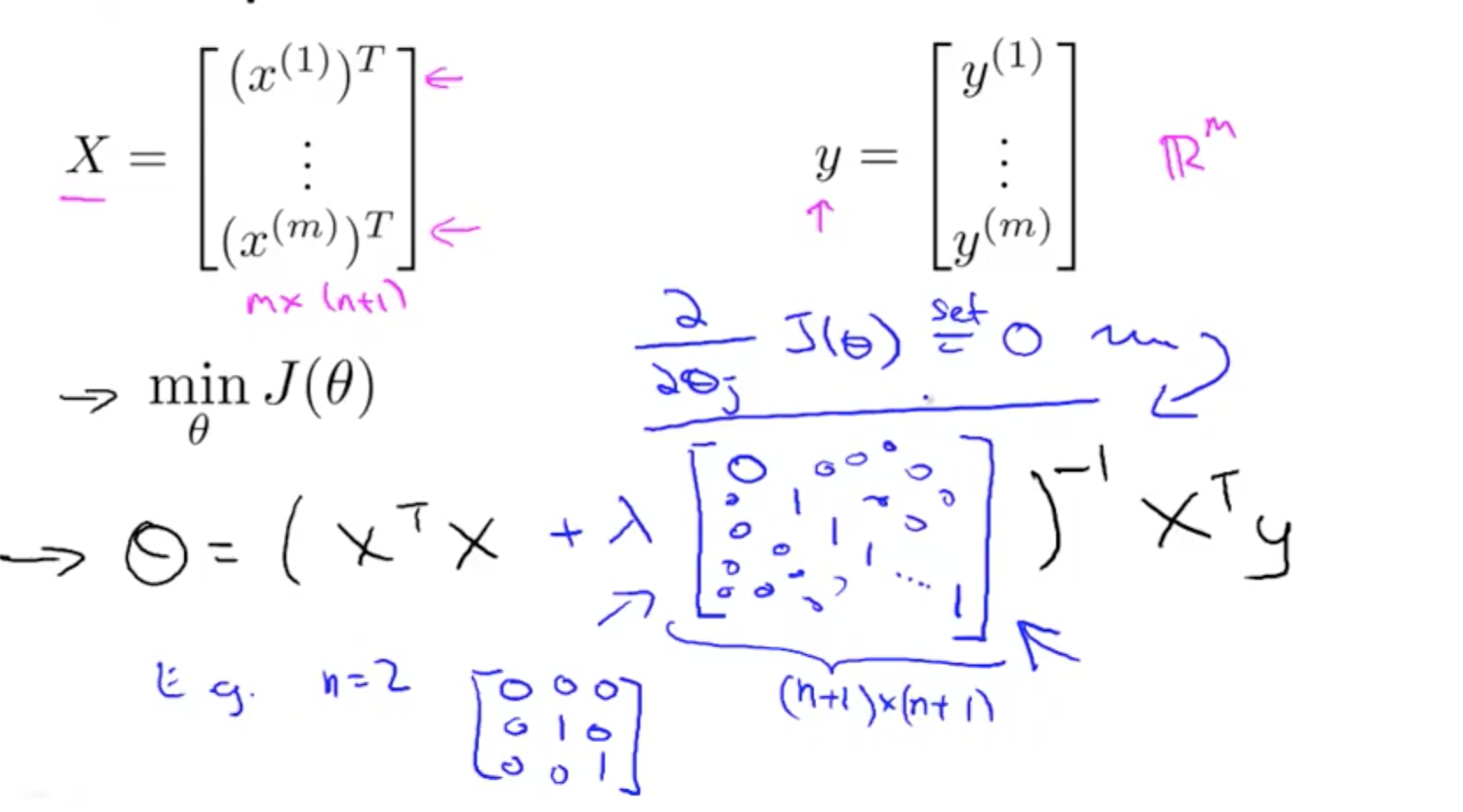• Non-invertibility
• Regularization takes care of non-invertibility
• Matrix will not be singular, it will be invertible

### 4c. Regularized Logistic Regression

• Cost function with regularization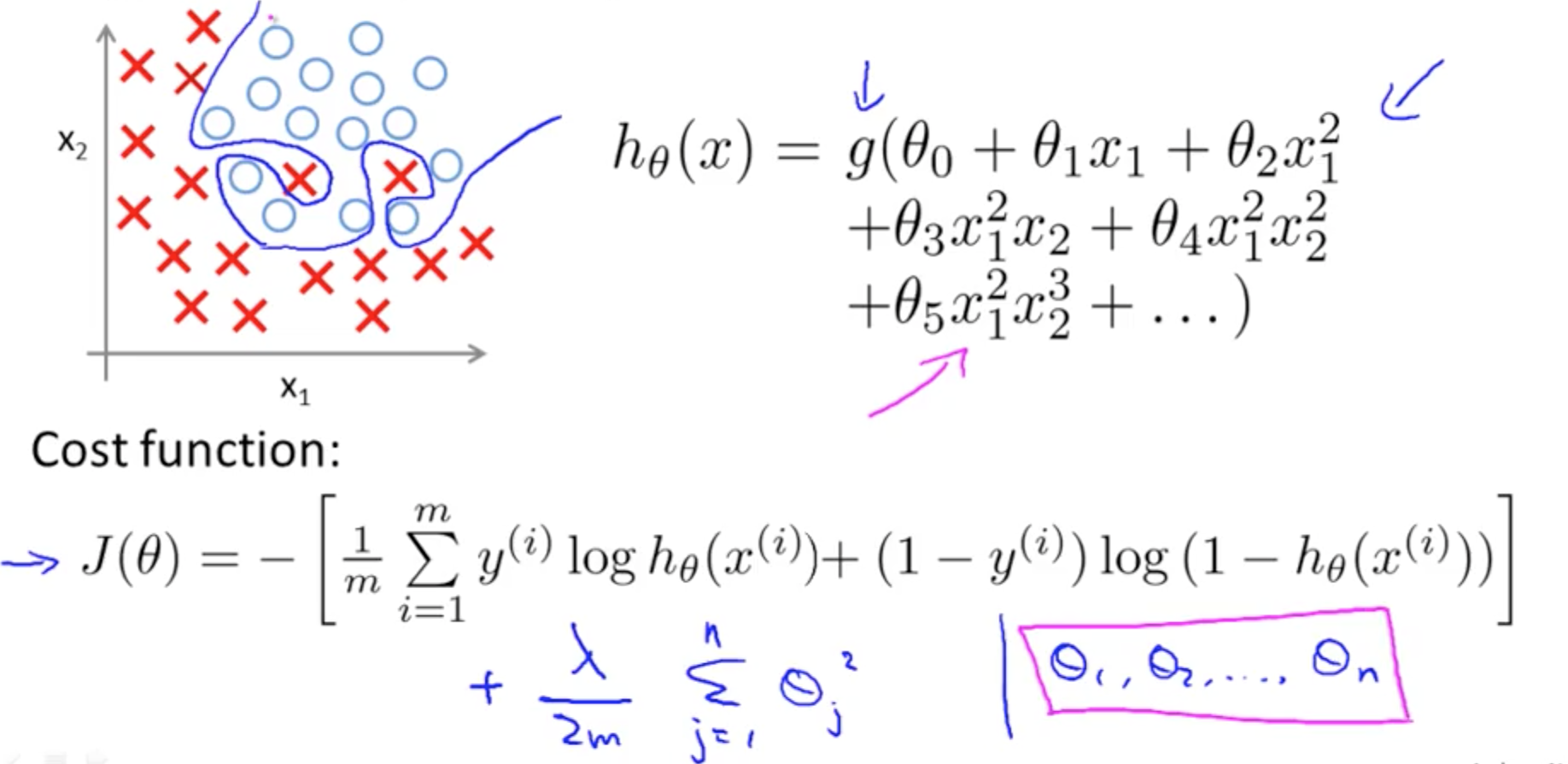• Using Gradient Descent for Regularized Logistic Regression Cost Function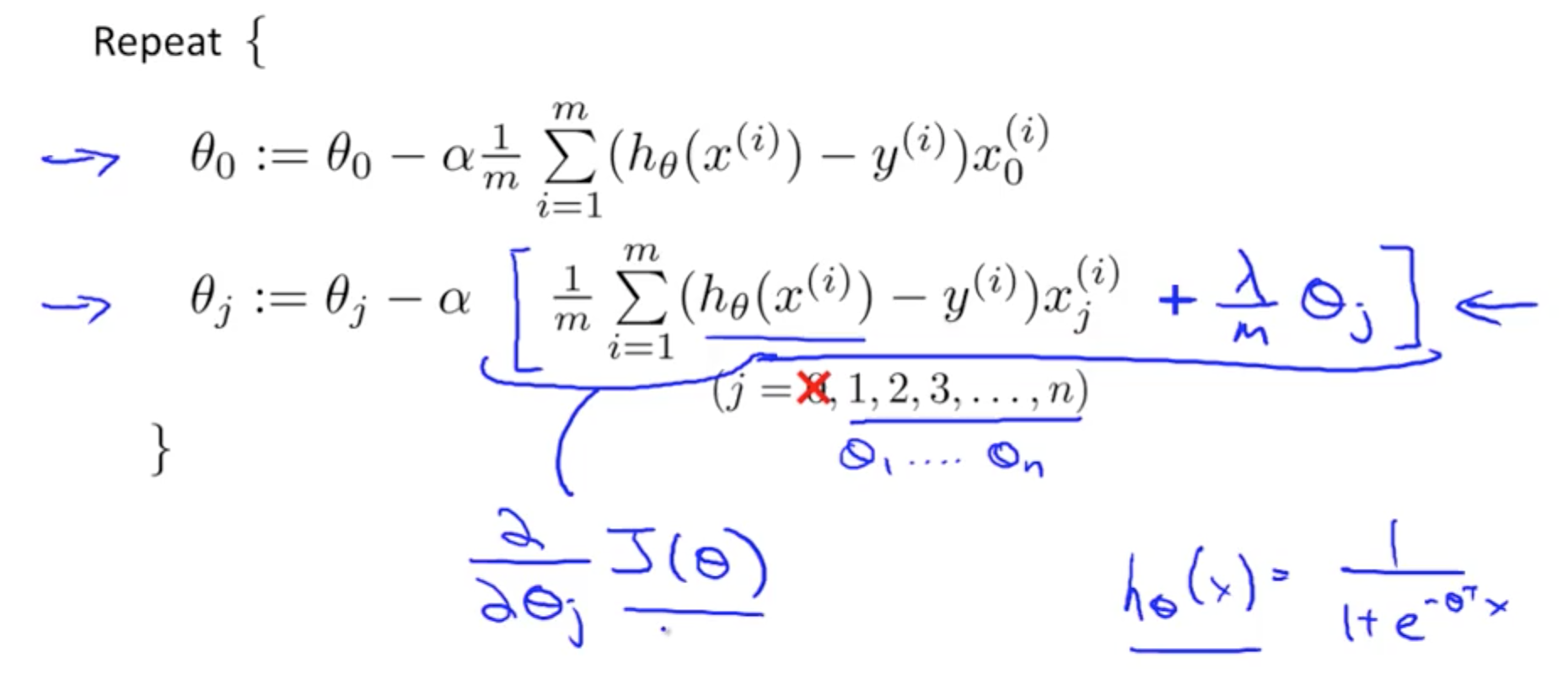• To check if Gradient Descent is working well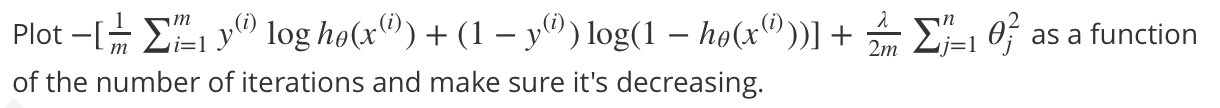• gradient